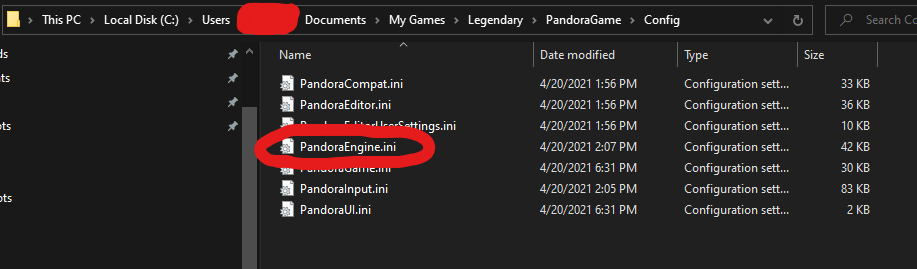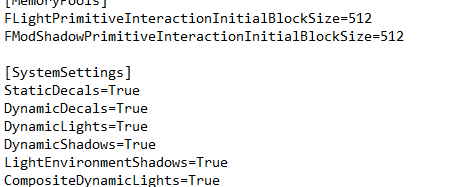# Legendary: How to Fix Graphical & Mouse Acceleration Issues 2021

This guide will hopefully help you fix the various issues the game currently has, it was mostly sourced from a discussion created by Scoob. I’ve tried other alternatives but this one seemed to work on my system, so I wanted to make this solution more easy to access for people who might need it. I’m trying to contact the OP about the guide and if they don’t want it up I’ll gladly take it down.

### Clay Textures and Low Resolution Issue

This section is for people having issues with their textures, resolution and overall graphical settings issues.

Step 1

In order to fix this issue you have to find your PandoraEngine.ini file which should be located in:Step 2

Open PandoraEngine.ini and try to find the title: [SystemSettings]Step 3

Once you find it you should delete everything under the title, and paste the following instead (excluding the title of course):

```[SystemSettings]
StaticDecals=True
DynamicDecals=True
DynamicLights=True
CompositeDynamicLights=True
DirectionalLightmaps=True
MotionBlur=True
DepthOfField=True
AmbientOcclusion=True
Bloom=True
UseHighQualityBloom=True
Distortion=True
DropParticleDistortion=True
SpeedTreeLeaves=True
SpeedTreeFronds=True
OnlyStreamInTextures=True
LensFlares=True
FogVolumes=True
FloatingPointRenderTargets=True
UseVsync=False
UpscaleScreenPercentage=True
Fullscreen=True
AllowD3D10=False
SkeletalMeshLODBias=0
ParticleLODBias=0
DetailMode=2
MaxAnisotropy=16
MaxMultisamples=4
ResX=1920
ResY=1080
ScreenPercentage=100.000000
SceneCaptureStreamingMultiplier=1.000000
bAllowFracturedDamage=True
NumFracturedPartsScale=1.000000
FractureDirectSpawnChanceScale=1.000000
FractureCullDistanceScale=1.000000
TEXTUREGROUP_World=(MinLODSize=256,MaxLODSize=1024,LODBias=0)
TEXTUREGROUP_WorldNormalMap=(MinLODSize=512,MaxLODSize=1024,LODBias=0)
TEXTUREGROUP_WorldSpecular=(MinLODSize=256,MaxLODSize=1024,LODBias=0)
TEXTUREGROUP_Character=(MinLODSize=512,MaxLODSize=1024,LODBias=0)
TEXTUREGROUP_CharacterNormalMap=(MinLODSize=512,MaxLODSize=1024,LODBias=0)
TEXTUREGROUP_CharacterSpecular=(MinLODSize=512,MaxLODSize=1024,LODBias=0)
TEXTUREGROUP_Weapon=(MinLODSize=512,MaxLODSize=1024,LODBias=0)
TEXTUREGROUP_WeaponNormalMap=(MinLODSize=1024,MaxLODSize=1024,LODBias=0)
TEXTUREGROUP_WeaponSpecular=(MinLODSize=512,MaxLODSize=1024,LODBias=0)
TEXTUREGROUP_Vehicle=(MinLODSize=256,MaxLODSize=1024,LODBias=1)
TEXTUREGROUP_VehicleNormalMap=(MinLODSize=256,MaxLODSize=1024,LODBias=1)
TEXTUREGROUP_VehicleSpecular=(MinLODSize=512,MaxLODSize=1024,LODBias=1)
TEXTUREGROUP_Cinematic=(MinLODSize=512,MaxLODSize=2048,LODBias=0)
TEXTUREGROUP_Effects=(MinLODSize=256,MaxLODSize=512,LODBias=0)
TEXTUREGROUP_Skybox=(MinLODSize=512,MaxLODSize=2048,LODBias=0)
TEXTUREGROUP_UI=(MinLODSize=1024,MaxLODSize=2048,LODBias=0)
TEXTUREGROUP_RenderTarget=(MinLODSize=512,MaxLODSize=2048,LODBias=0)
TEXTUREGROUP_SmallPropTexture=(MinLODSize=256,MaxLODSize=1024,LODBias=0)
TEXTUREGROUP_BigFlipBook=(MinLODSize=256,MaxLODSize=4096,LODBias=1)
TEXTUREGROUP_CharacterHigh=(MinLODSize=1024,MaxLODSize=1024,LODBias=0)
TEXTUREGROUP_CharacterLow=(MinLODSize=256,MaxLODSize=512,LODBias=0)
TEXTUREGROUP_CharacterNormalMapHigh=(MinLODSize=1024,MaxLODSize=1024,LODBias=0)
TEXTUREGROUP_CharacterNormalMapLow=(MinLODSize=256,MaxLODSize=512,LODBias=0)
TEXTUREGROUP_CharacterMini=(MinLODSize=256,MaxLODSize=256,LODBias=0)
TEXTUREGROUP_CharacterNormalMapMini=(MinLODSize=256,MaxLODSize=256,LODBias=0)
TEXTUREGROUP_WeaponHigh=(MinLODSize=1024,MaxLODSize=1024,LODBias=0)
TEXTUREGROUP_WeaponNormalMapHigh=(MinLODSize=1024,MaxLODSize=1024,LODBias=0)
TEXTUREGROUP_WeaponLow=(MinLODSize=256,MaxLODSize=512,LODBias=0)
TEXTUREGROUP_WeaponNormalMapLow=(MinLODSize=256,MaxLODSize=512,LODBias=0)
TEXTUREGROUP_WeaponMini=(MinLODSize=256,MaxLODSize=256,LODBias=0)
TEXTUREGROUP_WeaponNormalMapMini=(MinLODSize=256,MaxLODSize=256,LODBias=0)
TEXTUREGROUP_EffectsHigh=(MinLODSize=1024,MaxLODSize=1024,LODBias=0)
QualityBloom=True
Trilinear=True
EnableHighPolyChars=True```
Step 4 + BONUS

Now after doing the last step find the lines that have the settings “ResX” and “ResY” in the block of text we pasted and change them to your resolution or any resolution of your desire, it’s currently set to 1920×1080 which are my settings. If your computer starts lagging after these changes you may come back and change some of the settings from True to False, which is basically the equivalent of turning them on and off, their names are descriptive enough so I won’t talk about what each setting does.

You can also remove the intro movies by deleting the following lines from the PandoraEngine.ini file we’re in (they’re near the end of the file, if you’re too lazy to scroll down you can use Control + F):

```StartupMovies=LegalScreen_Final
StartupMovies=SparkLogo
StartupMovies=Logo_Gamecock_Final
StartupMovies=Logo_Atari_Final
StartupMovies=unreal_tech_movie
StartupMovies=gamespy
StartupMovies=amBX_logo_widescreen```

After you’ve done your changes, save everything exit the file and right click on it and select Properties. At the bottom of the General tab in Properties you can find the “Read only” option which stops the game from overwriting/modifying these settings we’ve made. Note that if you do this and decide to come back to change something you have to disable “Read only” before you do any changes.

If you own an NVIDIA GPU like I do, I had to change the settings for the game from “Integrated Graphics” to my actual GPU from the NVIDIA Control Panel for the game to run properly (you can look up how to access it and how to use it online).

### How to Disable Mouse Acceleration

Step 1

In order to fix this issue you have to find your PandoraInput.ini file which should be located in: